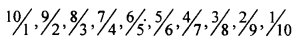# KSEEB Solutions for Class 8 Maths Chapter 7 Rational Numbers Ex 7.2

In this chapter, we provide KSEEB SSLC Class 8 Maths Chapter 7 Rational Numbers Ex 7.2 for English medium students, Which will very helpful for every student in their exams. Students can download the latest KSEEB SSLC Class 8 Maths Chapter 7 Rational Numbers Ex 7.2 pdf, free KSEEB SSLC Class 8 Maths Chapter 7 Rational Numbers Ex 7.2 pdf download. Now you will get step by step solution to each question.

## Karnataka Board Class 8 Maths Chapter 7 Rational Numbers Ex 7.2

Question 1.
Write down ten rational numbers which are equivalent to 57 and the denominator not exceeding 80.
Multiply both numerator and denominator by 2, 3, 4………
1014,1521,2028,3535,3042,3549,4056,4563,5070,5577

Question 2.
Write down 15 rational numbers which are equivalent to 115 and the numerator not exceeding 180.
2210,3315,4420,5525,6630,7735,8840,994511050,12155,13260,14365,15470,16575,17680

Question 3.
Write down 10 positive rational numbers such that the sum of the numerator and the denominator of each is 11. Write them in decreasing order.Question 4.
Write down ten positive rational numbers such that numerator – denominator for each of them is -2. Write to them in increasing order.
Numerator – denominator = – 2
therefore the denominator is greater than the numerator by 2.
13,24,35,46,57,69,79,810,911,1012

Question 5.
Is 3−2 a rational number? If so, how do you write it in the form conforming to the definition of a rational number (that is, the denominator as positive integer)?
3−2 is a rational number because the denominator is negative.
It can be written as 3−2 since 3−2 is same as 3−2

Question 6.
Earlier you have studied decimals 0.9, 0.8, can you’ write these as rational numbers?
0.9=910 and 0.8=810=45

All Chapter KSEEB Solutions For Class 8 maths

—————————————————————————–

All Subject KSEEB Solutions For Class 9

*************************************************

I think you got complete solutions for this chapter. If You have any queries regarding this chapter, please comment on the below section our subject teacher will answer you. We tried our best to give complete solutions so you got good marks in your exam.

If these solutions have helped you, you can also share kseebsolutionsfor.com to your friends.

Best of Luck!!